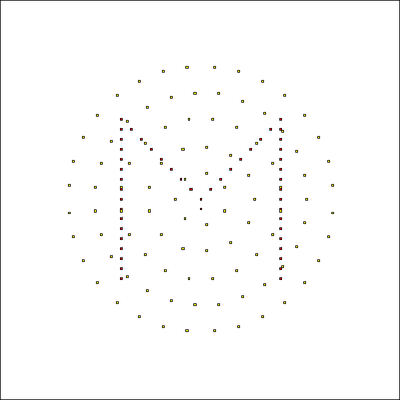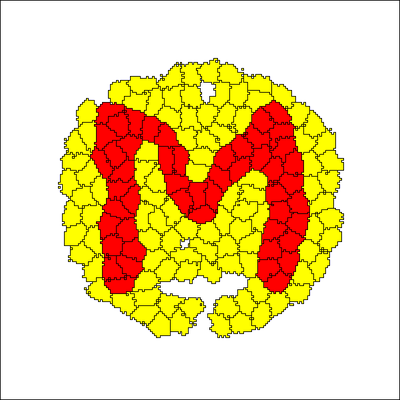# Initialize cell population from CSV

##### Persistent Identifier

This model also requires the separate file Population_M.csv with the list of lattice nodes where cells shall be initialized.

## Introduction

Initializing the spatial configuration of cells is a key step of every simulation and Morpheus offers all the flexibility for this:

1. the model section CellPopulations/Population (in the GUI and xml) can declare geometrical rules incl.
• InitCellObjects/Arrangement (cell repetitions in (x,y,z), displacements in (x,y,z) and random_displacement) of different cell shapes with adjustable sizes and rotation angles (Point, Box, Sphere, Ellipsoid, Cylinder)
• InitCircle (seed cells in a circular subdomain), InitDistribute, InitHexLattice, InitPoissonDisc, InitRectangle, InitVoronoi
1. you can overlay multiple CellPopulations/Population groups with the above constructs
2. all these arrangements together can be masked by Lattice/Domain
3. you may specify the initial cell shapes or just set single points that will inflate into cell shapes during an initial transient of the model simulation
4. you can load a CellPopulations/Population from files, both CSV (for lists of nodes for seeding as shown below) or TIFF (for pixel-based spatial layout where pixel color is interpreted as CellID, so you can segment from microscopy images or draw arbitrary areas each with the same color)
5. you can run a helper model that distributes the cells as you need and saves a snapshot of the spatial configuration with Time/SaveInterval (then load the snapshot model.xml and copy/paste its CellPopulations/Population from the snapshot model to your main model)

All these rules for initializing the spatial configuration of cells are included in the same xml file as the model rules. So you can reproduce the exact same initialization from the model.xml.

For a realistic epithelial cell arrangement, see this Blog post.

## Description

Here we use the file Population_M.csv with a list of seeding positions to initialize one of two cell populations with the shape of the letter M.Snapshot at time=0 with the loaded single nodes forming an M for the (red) cell population superimposed on another randomly placed set of nodes for the (yellow) cell population.Snapshot at time=100, color code as described for previous figure.

The video shows how the single nodes inflate to cell shapes during 100 Monte Carlo steps.

## Model

Get this model via:

•  Download: Population_M.xml
• XML Preview

<MorpheusModel version="4">
<Description>
<Title>Example-CellSorting-2D</Title>
<Details>Demo of cell population initialization from a file (here Population_M.csv).
</Details>
</Description>
<Global/>
<Space>
<SpaceSymbol symbol="l"/>
<Lattice class="square">
<Size symbol="size" value="200, 200, 0"/>
<BoundaryConditions>
<Condition type="periodic" boundary="x"/>
<Condition type="periodic" boundary="y"/>
</BoundaryConditions>
<Neighborhood>
<Order>2</Order>
</Neighborhood>
</Lattice>
</Space>
<Time>
<StartTime value="0"/>
<StopTime value="100"/>
<RandomSeed value="0"/>
<TimeSymbol symbol="time"/>
</Time>
<CellTypes>
<CellType name="ct1" class="biological">
<VolumeConstraint target="100" strength="1"/>
</CellType>
<CellType name="ct2" class="biological">
<VolumeConstraint target="100" strength="1"/>
</CellType>
<CellType name="medium" class="medium"/>
</CellTypes>
<CPM>
<Interaction default="0.0">
<Contact type2="medium" type1="ct1" value="12"/>
<Contact type2="medium" type1="ct2" value="6"/>
<Contact type2="ct1" type1="ct1" value="6"/>
<Contact type2="ct2" type1="ct2" value="6"/>
<Contact type2="ct2" type1="ct1" value="16"/>
</Interaction>
<MonteCarloSampler stepper="edgelist">
<MCSDuration value="1"/>
<Neighborhood>
<Order>2</Order>
</Neighborhood>
<MetropolisKinetics temperature="2"/>
</MonteCarloSampler>
<ShapeSurface scaling="norm">
<Neighborhood>
<Order>6</Order>
</Neighborhood>
</ShapeSurface>
</CPM>
<CellPopulations>
<Population type="ct1" size="0">
</Population>
<Population type="ct2" size="0">
<InitCircle mode="regular" number-of-cells="100">
</InitCircle>
</Population>
</CellPopulations>
<Analysis>
<Gnuplotter time-step="10" decorate="false">
<Terminal name="png"/>
<Plot>
<Cells flooding="true" value="cell.type"/>
</Plot>
</Gnuplotter>
<ModelGraph include-tags="#untagged" format="dot" reduced="false"/>
</Analysis>
</MorpheusModel>



This model also requires the separate file Population_M.csv.